# AP Physics 1 Multiple-Choice Practice Test 23

### Test Information10 questions18 minutes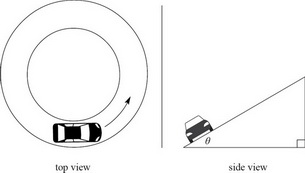1. A car is traveling in a horizontal circle of radius r, on a banked turn, at a constant speed v. How does the magnitude of the normal force on the car compare with the car's weight?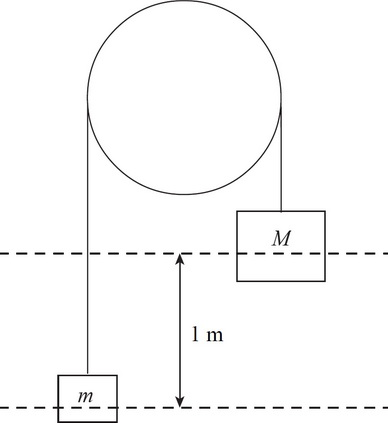2. The pulley above is both frictionless and massless, and the blocks are initially at rest. How long will it take for the two blocks to reach an equal height if block M of mass 15 kg starts 1 m above block m of mass 5 kg?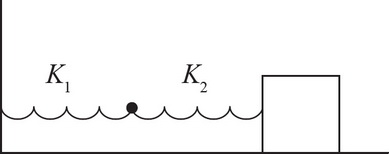3. The spring-block system above has a block of mass m oscillating in simple harmonic motion. However, instead of one spring, there are two springs connected to each other. The first spring has a spring constant k1, and the second has a spring constant k2. What would be the effective spring constant of the system above if k2 = 3k1 ?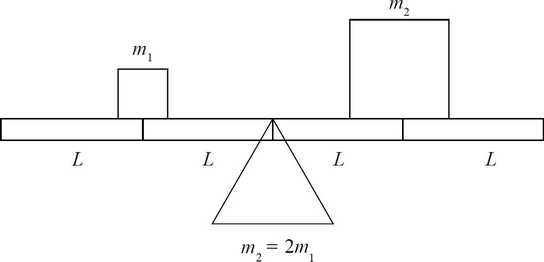4. As it is, the system above is not balanced. Which of the following changes would NOT balance the system so that there is 0 net torque? Assume the plank has no mass of its own.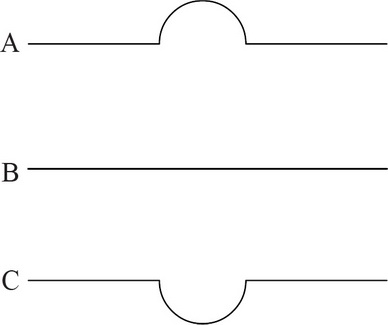5. Three identical balls are rolled from left to right across the three tracks above with the same initial speed. Assuming the tracks all have negligible friction and the balls have enough initial speed to reach the ends of each track, which set correctly orders the average speed of the balls on the three tracks?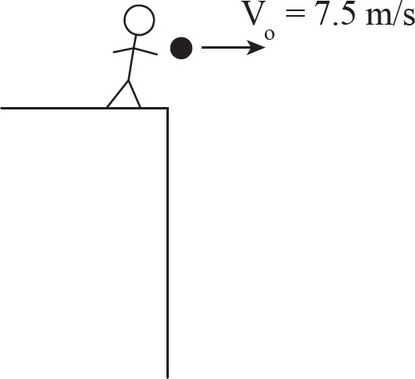6. An object is thrown horizontally to the right off a high cliff with an initial speed of 7.5 m/s. Which arrow best represents the direction of the object's velocity after 2 seconds? (Assume air resistance is negligible.)

7. Question below refers to the following figure: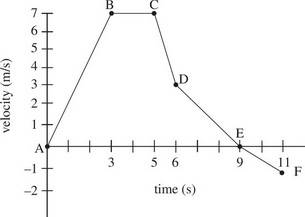Rank the change in momentum for each segment from least (most negative) to greatest (most positive). Assume that the mass of the object remains constant.

8. Question below refers to the following figure:During which segment is the magnitude of average acceleration greatest?

9. Question below refers to the following figure:What is the total distance traveled by the object?10. A ball is thrown with an initial velocity v at an angle θ above the ground. If the acceleration due to gravity is -g, which of the following is the correct expression of the time it takes for the ball to reach its highest point, y, from the ground?